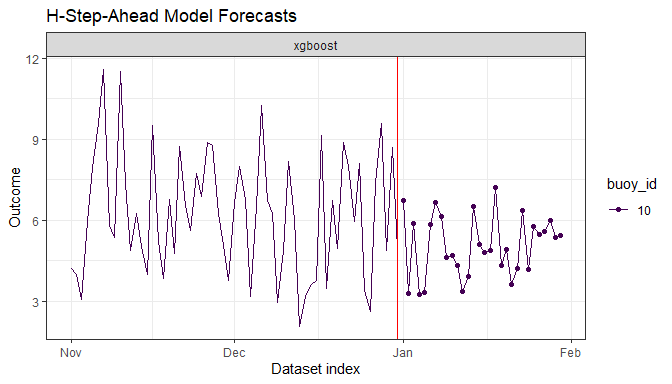# Purpose

The purpose of this vignette is to provide an overview of forecasting with multiple time series in forecastML. The benefits to modeling multiple time series in one go with a single model or ensemble of models include (a) modeling simplicity, (b) potentially more robust results from pooling data across time series, and (c) solving the cold-start problem when few data points are available for a given time series.

We won’t concern ourselves with error metrics or hyperparameter evaluation which can be assessed with the return_error() and return_hyper() functions. This functionality is covered in the package overview vignette.

# Setup

To forecast with multiple/grouped/hierarchical time series in forecastML, your data need the following characteristics:

• The same outcome is being forecasted across time series.

• Data are in a long format with a single outcome column–i.e., time series are stacked on top of each other in a data.frame.

• There are 1 or more grouping columns.

• There may be 1 or more static features that are constant through time but differ between time series–e.g., a fixed location, store square footage, species of animal etc.

• The time series are regularly spaced and have no missing rows or gaps in time. Irregular or sparse time series with many NAs can be modeled in this framework, but missing rows will result in incorrect feature lags when using create_lagged_df() which is the first step in the forecastML workflow. To fix any gaps in data collection, use the fill_gaps() function. Handling the resulting missing values in the target being forecasted and any dynamic features can be done (a) prior to create_lagged_df() or (b) in the user-defined model training function.

# Example

To illustrate forecasting with multiple time series, we’ll use the data_buoy dataset that comes with the package. This dataset consists of daily sensor measurements of several environmental conditions collected by 14 buoys in Lake Michigan from 2012 through 2018. The data were obtained from NOAA’s National Buoy Data Center available at https://www.ndbc.noaa.gov/ using the rnoaa package.

• Outcome: Average daily wind speed in Lake Michigan.

• Forecast horizon: Daily, 1 to 30 days into the future which is essentially January 2019 for this dataset.

• Time series: 14 outcome time series collected from buoys throughout Lake Michigan.

• Model: A single gradient boosted tree model with xgboost for each of 3 direct forecast horizons.

## Load Packages and Data

data_buoy_gaps consists of:

• date: A date column which will be removed for modeling.

• wind_spd: The outcome which is treated as a lagged feature by default.

• lat and lon: Latitude and longitude which are features that are static or unchanging through time.

• day and year: Dynamic features which won’t be lagged but whose future values will be filled in when forecasting.

• air_temperature and sea_surface_temperature: Data collected from the buoys through time (lagged features).

library(dplyr)
library(DT)
library(ggplot2)
library(forecastML)
library(xgboost)

data("data_buoy_gaps", package = "forecastML")

DT::datatable(head(data_buoy_gaps), options = list(scrollX = TRUE))

## forecastML::fill_gaps

• The wind speed data has some gaps in it: Some buoys collected data throughout the year, others only during the summer months. These gaps in data collection would result in incorrect feature lags in create_lagged_df() as the previous row in the dataset for a given buoy–a lag of 1–may be several months in the past.

• To fix this problem, we’ll run fill_gaps() to fill in the rows for the missing dates. The added rows will appear between min(date) for each buoy and max(date) across all buoys. For example, buoy 45186 that only started data collection in 2018 won’t have additional rows with NAs for 2012 through 2017; only gaps since the start of data collection in 2018 to the most recent date will be filled in.

• After running fill_gaps(), the following columns have been filled in and have no NAs: date, buoy_id, lat, and lon.

• After running fill_gaps(), the following columns now have additional NAs: our wind_spd target and the dynamic features.

• Notice that the input dataset and the returned dataset have the same columns in the same order with the same data types.

data <- forecastML::fill_gaps(data_buoy_gaps, date_col = 1, frequency = '1 day',
groups = 'buoy_id', static_features = c('lat', 'lon'))

print(list(paste0("The original dataset with gaps in data collection is ", nrow(data_buoy_gaps), " rows."),
paste0("The modified dataset with no gaps in data collection from fill_gaps() is ", nrow(data), " rows.")))
## []
##  "The original dataset with gaps in data collection is 23646 rows."
##
## []
##  "The modified dataset with no gaps in data collection from fill_gaps() is 31225 rows."

## Dynamic Features

• Now would be a good time to fill in the newly created missing values in our dynamic features: day and year. These features are deterministic and won’t be lagged in the modeling dataset. We could also impute missing values for air_temperature and sea_surface_temperature, but we’ll let our xgboost model handle these NAs.
data$day <- lubridate::mday(data$date)
data$year <- lubridate::year(data$date)

## Plot Wind Speed Outcome

• Notice that buoy 45186 has only recently come online and would be difficult to forecast on its own.
p <- ggplot(data, aes(x = date, y = wind_spd, color = ordered(buoy_id), group = year))
p <- p + geom_line()
p <- p + facet_wrap(~ ordered(buoy_id), scales = "fixed")
p <- p + theme_bw() + theme(
legend.position = "none"
) + xlab(NULL)
p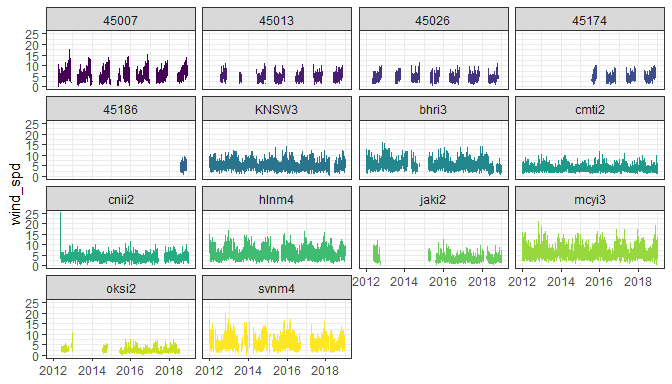## Modeling Setup

• We’ll simply and incorrectly set our grouping column, buoy_id, to numeric to work smoothly with xgboost. Better alternatives include feature embedding, target encoding (available in the R package catboost), or mixed effects Random Forests.

• To be clear, buoy_id is both (a) used to identify a specific time series for creating lagged features and (b) used as a feature in the model.

data$buoy_id <- as.numeric(factor(data$buoy_id))
• Inputs for dataset creation with create_lagged_df().
outcome_col <- 1  # The column position of our 'wind_spd' outcome.

horizons <- c(1, 7, 30)  # Forecast 1, 1:7, and 1:30 days into the future.

lookback <- c(1:30, 360:370)  # Features from 1 to 30 days in the past and annually.

dates <- data$date # Grouped time series forecasting requires dates. data$date <- NULL  # Dates, however, don't need to be in the input data.

frequency <- "1 day"  # A string that works in base::seq(..., by = "frequency").

dynamic_features <- c("day", "year")  # Features that change through time but which will not be lagged.

groups <- "buoy_id"  # 1 forecast for each group or buoy.

static_features <- c("lat", "lon")  # Features that do not change through time.

## Model Training with Nested CV

### Training dataset

• We have 3 datasets for training models that forecast 1, 1 to 7, and 1 to 30 days into the future. We’ll view the 1-day-ahead training data below.
type <- "train"  # Create a model-training dataset.

data_train <- forecastML::create_lagged_df(data, type = type, outcome_col = outcome_col,
horizons = horizons, lookback = lookback,
dates = dates, frequency = frequency,
dynamic_features = dynamic_features,
groups = groups, static_features = static_features,
use_future = FALSE)

DT::datatable(head(data_train$horizon_1), options = list(scrollX = TRUE)) • The plot below shows the feature map for any lagged features across forecast horizons. Here, we set all non-dynamic and non-static features to have the same lags (refer to the custom lags vignette to see how this could be modified). Notice that features that don’t support direct forecasting to the given horizon–e.g., lags of 1 to 29 days for the 30-day-horizon model–are silently dropped. p <- plot(data_train) # plot.lagged_df() returns a ggplot object. p <- p + geom_tile(NULL) # Remove the gray border for a cleaner plot. p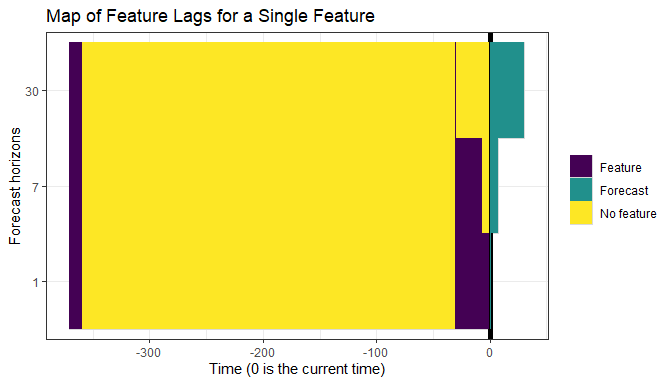### CV setup • We’ll model with 3 validation datasets. Given that our measurements are taken daily, we’ll set the skip = 730 argument to skip 2 years between validation datasets. Custom validation windows could be defined with vectors of start and stop dates given to window_start and window_stop. windows <- forecastML::create_windows(data_train, window_length = 365, skip = 730, include_partial_window = FALSE) p <- plot(windows, data_train) + theme(legend.position = "none") p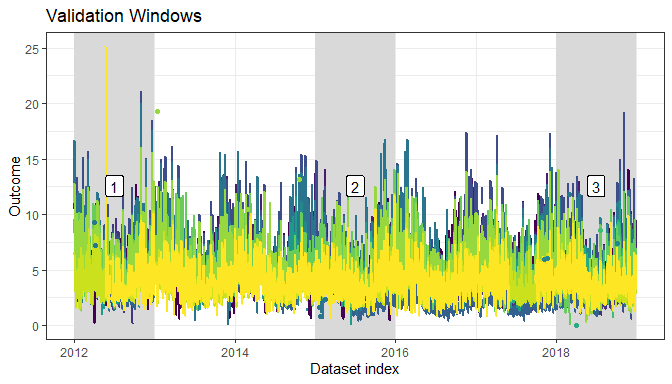• Now we’ll use the group_filter = "buoy_id == 1" argument to get a closer look at 1 of our 14 time series. The user-supplied filter is passed to dplyr::filter() internally. p <- plot(windows, data_train, group_filter = "buoy_id == 1") + theme(legend.position = "none") p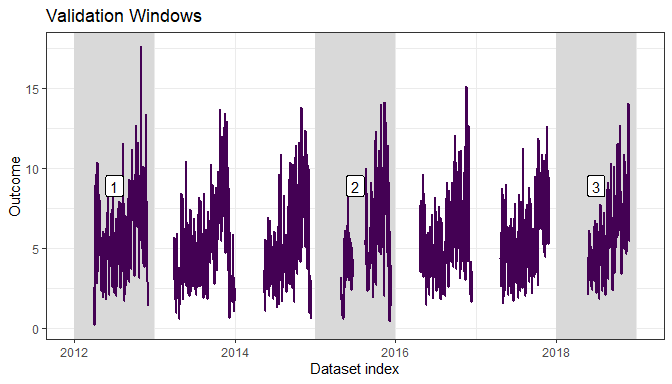### User-defined modeling function • A user-defined wrapper function for model training that takes the following arguments: • 1: A horizon-specific data.frame made with create_lagged_df(..., type = "train") (e.g., my_lagged_df$horizon_h),
• 2: optionally, any number of additional named arguments which can be passed as ‘…’ in train_model()
• and returns a model object or list containing a model that will be passed into the user-defined predict() function.

Any data transformations, hyperparameter tuning, or inner loop cross-validation procedures should take place within this function, with the limitation that it ultimately needs to return() a model suitable for the user-defined predict() function; a list can be returned to capture meta-data such as pre-processing pipelines or hyperparameter results.

• Notice that the xgboost-specific input datasets are created within this wrapper function.
# The value of outcome_col can also be set in train_model() with train_model(outcome_col = 1).
model_function <- function(data, outcome_col = 1) {

# xgboost cannot handle missing outcomes data so we'll remove this.
data <- data[!is.na(data[, outcome_col]), ]

indices <- 1:nrow(data)

set.seed(224)
train_indices <- sample(1:nrow(data), ceiling(nrow(data) * .8), replace = FALSE)
test_indices <- indices[!(indices %in% train_indices)]

data_train <- xgboost::xgb.DMatrix(data = as.matrix(data[train_indices,
-(outcome_col), drop = FALSE]),
label = as.matrix(data[train_indices,
outcome_col, drop = FALSE]))

data_test <- xgboost::xgb.DMatrix(data = as.matrix(data[test_indices,
-(outcome_col), drop = FALSE]),
label = as.matrix(data[test_indices,
outcome_col, drop = FALSE]))

params <- list("objective" = "reg:linear")

watchlist <- list(train = data_train, test = data_test)

set.seed(224)
model <- xgboost::xgb.train(data = data_train, params = params,
max.depth = 8, nthread = 2, nrounds = 30,
metrics = "rmse", verbose = 0,
early_stopping_rounds = 5,
watchlist = watchlist)

return(model)
}

### Model training

• This should take ~1 minute to train our ‘3 forecast horizons’ * ‘3 validation datasets’ = 9 models.

• The user-defined modeling wrapper function could be much more elaborate, in which case many more models could potentially be trained here.

• These models could be trained in parallel on any OS with the very flexible future package by uncommenting the code below and setting use_future = TRUE. To avoid nested parallelization, models are either trained in parallel across forecast horizons or validation windows, whichever is longer (when equal, the default is parallel across forecast horizons).

#future::plan(future::multiprocess)  # Multi-core or multi-session parallel training.

model_results_cv <- forecastML::train_model(lagged_df = data_train,
windows = windows,
model_name = "xgboost",
model_function = model_function,
use_future = FALSE)
• We can access the xgboost model for any horizon or validation window. Here, we show a summary() of the model for the first validation window which is 2012.
summary(model_results_cv$horizon_1$window_1$model) ## Length Class Mode ## handle 1 xgb.Booster.handle externalptr ## raw 308719 -none- raw ## best_iteration 1 -none- numeric ## best_ntreelimit 1 -none- numeric ## best_score 1 -none- numeric ## niter 1 -none- numeric ## evaluation_log 3 data.table list ## call 10 -none- call ## params 5 -none- list ## callbacks 2 -none- list ## feature_names 128 -none- character ## nfeatures 1 -none- numeric ## Forecasting with Nested Models • First, we’ll visually evaluate our model’s performance across our validation datasets. • Then we’ll forecast with each of our 9 models to get a sense of the stability of the forecasts produced from models trained on different subsets of our historical data. ### User-defined prediction function The following user-defined prediction function is needed for each model: • A wrapper function that takes the following 2 positional arguments: • 1: The model returned from the user-defined modeling function (could be a list containing the model). • 2: A data.frame of the model features from forecastML::create_lagged_df(..., type = "train"). • and returns a data.frame of predictions with 1 or 3 columns. A 1-column data.frame will produce point forecasts, and a 3-column data.frame can be used to return point, lower, and upper forecasts (column names and order do not matter). # If 'model' is passed as a named list, the prediction model would be accessed with model$model or model["model"].
prediction_function <- function(model, data_features) {
x <- xgboost::xgb.DMatrix(data = as.matrix(data_features))
data_pred <- data.frame("y_pred" = predict(model, x))
return(data_pred)
}

### Historical model fit

• Here, we’re predicting on our validation datasets.
data_pred_cv <- predict(model_results_cv, prediction_function = list(prediction_function), data = data_train)
• The predictions are solid lines; the actuals are dashed lines.

• We’ll filter this plot for closer inspection below.

plot(data_pred_cv) + theme(legend.position = "none")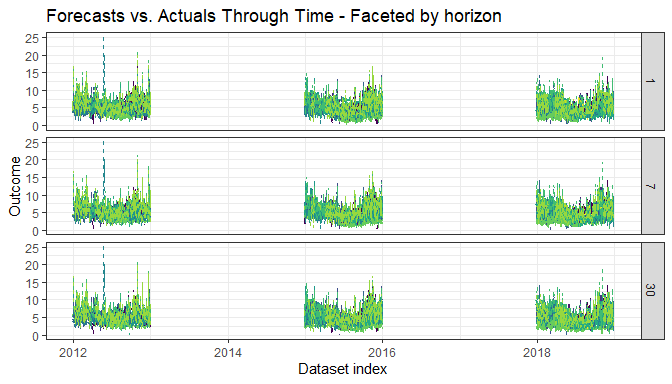• It’s somewhat difficult to see how we’ve done here, so we’ll use the group_filter argument again to focus on specific results. Note that the plot() function returns a ggplot object so that we can easily modify our plot.

• Notice that during the first half of 2015 there were no wind speed measurements recorded; however, we’re getting some interesting variability in predictions here because the day of the month and year dynamic features have captured some signal.

p <- plot(data_pred_cv, group_filter = "buoy_id %in% c(1, 2, 3)", horizons = 7)
p <- p + theme(legend.position = "none")
p <- p + scale_x_date(limits = c(as.Date("2015-01-01"), as.Date("2015-12-31")))
p <- p + facet_grid(horizon ~ buoy_id) + ggtitle(paste0(p$labels$title, " and buoy ID"))
p <- p + theme(axis.text.x = element_text(angle = 90))
p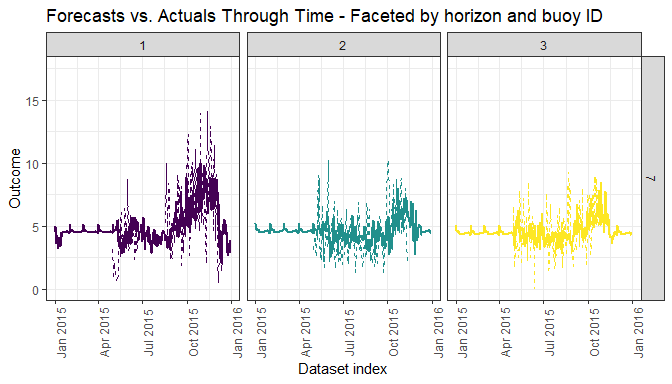### Forecasting

• We have 3 datasets that support forecasting 1, 1 to 7, and 1 to 30 days into the future. We’ll view the 1-day-ahead forecasting data below.

• Note that the index and horizon columns are removed internally when passed into the user-defined predict() function.

type <- "forecast"  # Create a forecasting dataset for our predict() function.

data_forecast <- forecastML::create_lagged_df(data, type = type, outcome_col = outcome_col,
horizons = horizons, lookback = lookback,
dates = dates, frequency = frequency,
dynamic_features = dynamic_features,
groups = groups, static_features = static_features,
use_future = FALSE)

DT::datatable(head(data_forecast$horizon_1), options = list(scrollX = TRUE)) ### Dynamic features and forecasting • Our dynamic features day and year were not lagged in our modeling dataset. This was the right choice from a modeling perspective; however, in order to forecast ‘h’ steps ahead, we need to know their future values for each forecast horizon. At present, there’s no function in forecastML to autofill the future values of dynamic, non-lagged features so we’ll simply do it manually below. for (i in seq_along(data_forecast)) { data_forecast[[i]]$day <- lubridate::mday(data_forecast[[i]]$index) # When dates are given, the 'index is date-based. data_forecast[[i]]$year <- lubridate::year(data_forecast[[i]]\$index)
}

### Forecast

• Now we’ll forecast 1, 1:7, and 1:30 days into the future with predict(..., data = data_forecast).

• The first time step into the future is max(dates) + 1 * frequency. Here, this is 12-31-2018 + 1 * ‘1 day’ or 1-1-2019.

data_forecasts <- predict(model_results_cv, prediction_function = list(prediction_function), data = data_forecast)
• Plots for each model–just xgboost here–and time horizon. The separate lines are for the combination of buoy_id and validation window.
plot(data_forecasts) + theme(legend.position = "none")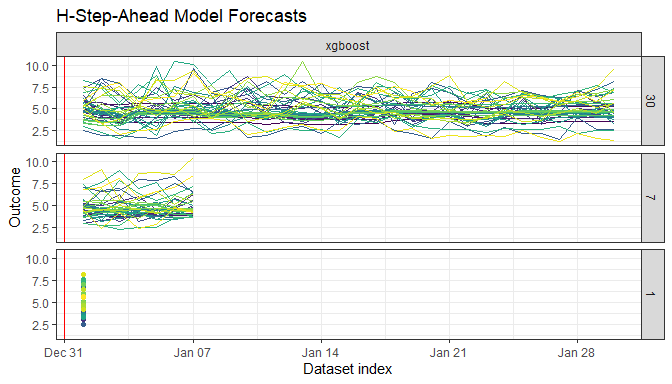• We’ll filter results to look at the forecasts across validation windows for buoy_id == 1. In this case, a validation window shows how the model would forecast if it were not trained on data in a given time frame or window.
plot(data_forecasts, group_filter = "buoy_id == 1")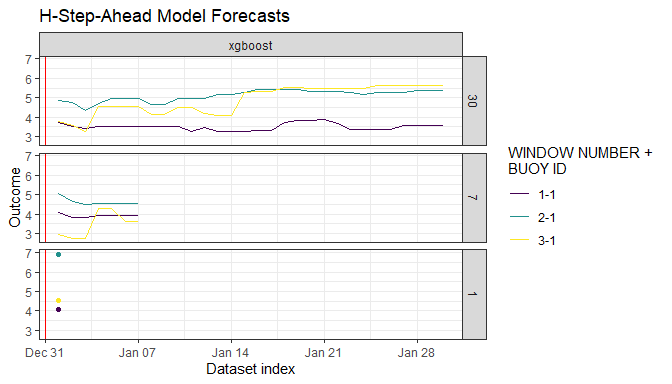## Model Training with All Data

• The modeling steps are more or less the same as in the nested cross-validation modeling described above so we’ll skip the explanations from here on out.

• Notice that by this point in the modeling process, the optimal hyperparameters that gave the best performance on the outer-loop validation datasets have already been identified (see the package overview vignette). Incorporating the optimal hyperparameters in a final model would occur in a new user-defined modeling wrapper function.

• Train across all data by setting window_length = 0.

windows <- forecastML::create_windows(data_train, window_length = 0)

p <- plot(windows, data_train) + theme(legend.position = "none")
p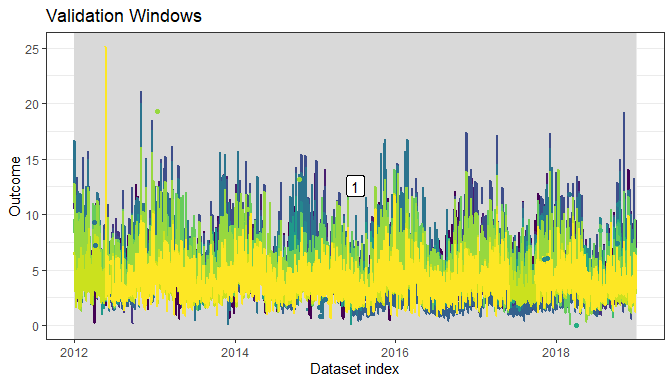# Un-comment the code below and set 'use_future' to TRUE.
#future::plan(future::multiprocess)

model_results_no_cv <- forecastML::train_model(lagged_df = data_train,
windows = windows,
model_name = "xgboost",
model_function = model_function,
use_future = FALSE)
data_forecasts <- predict(model_results_no_cv, prediction_function = list(prediction_function), data = data_forecast)

DT::datatable(head(data_forecasts), options = list(scrollX = TRUE))
plot(data_forecasts)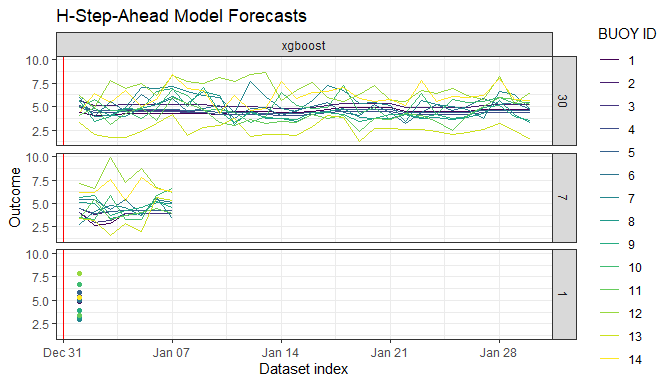## Forecast Combination

• The final step in the forecastML framework is to combine multiple direct-horizon forecast models with combine_forecasts() to produce a single h-step-ahead forecast.

• The default approach, type = 'horizon', is to combine forecasts across models such that short-term models produce the shorter-term forecasts and long-term models produce the longer-term forecasts. This implies that, for our 30-day-ahead forecast,

• The 1-step-ahead model forecasts the next day,
• The 7-step-ahead model forecasts from days 2 through 7, and
• The 30-step-ahead model forecasts from days 8 through 30.
• Plot all forecasts.

data_combined <- forecastML::combine_forecasts(data_forecasts)

# Plot a background dataset of actuals using the most recent data.
data_actual <- data[dates >= as.Date("2018-11-01"), ]
actual_indices <- dates[dates >= as.Date("2018-11-01")]

# Plot all final forecasts plus historical data.
plot(data_combined, data_actual = data_actual, actual_indices = actual_indices)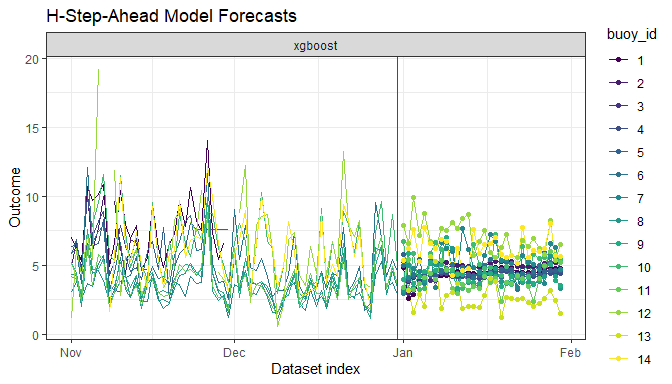• Plot a single forecast.
# Plot final forecasts for a single buoy plus historical data.
plot(data_combined, data_actual = data_actual, actual_indices = actual_indices,
group_filter = "buoy_id == 10")`